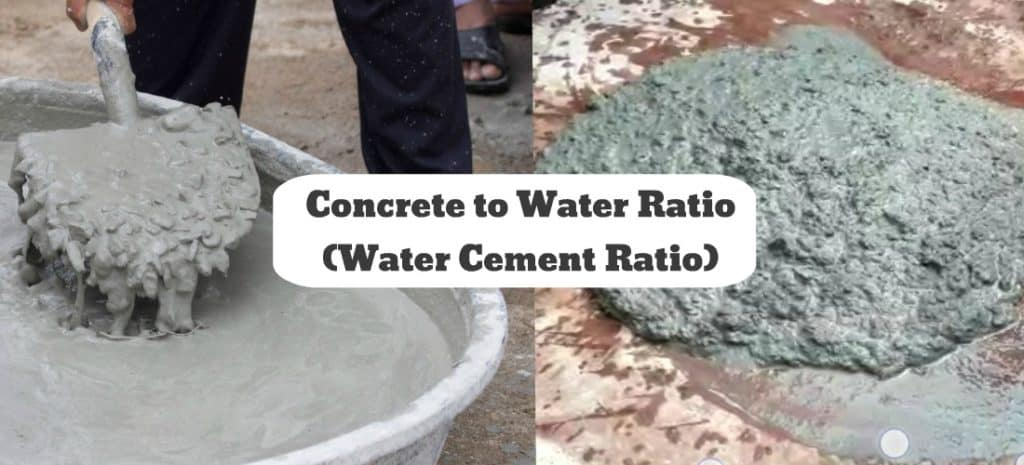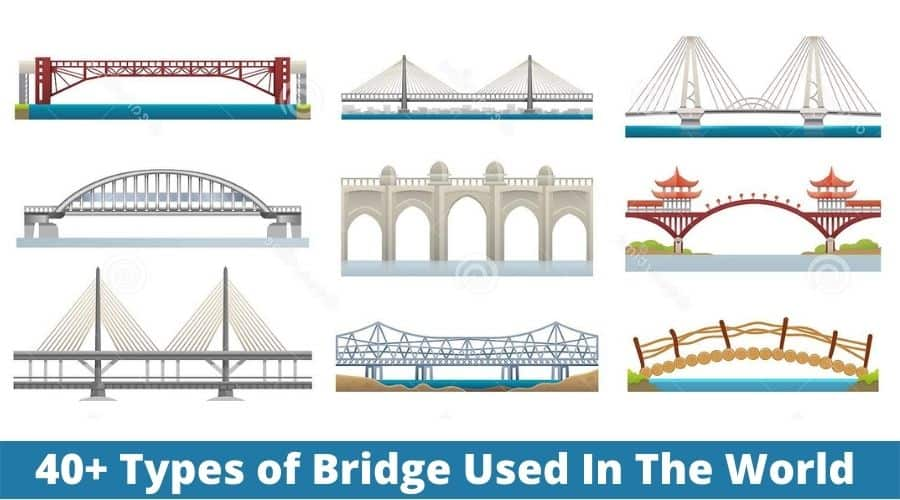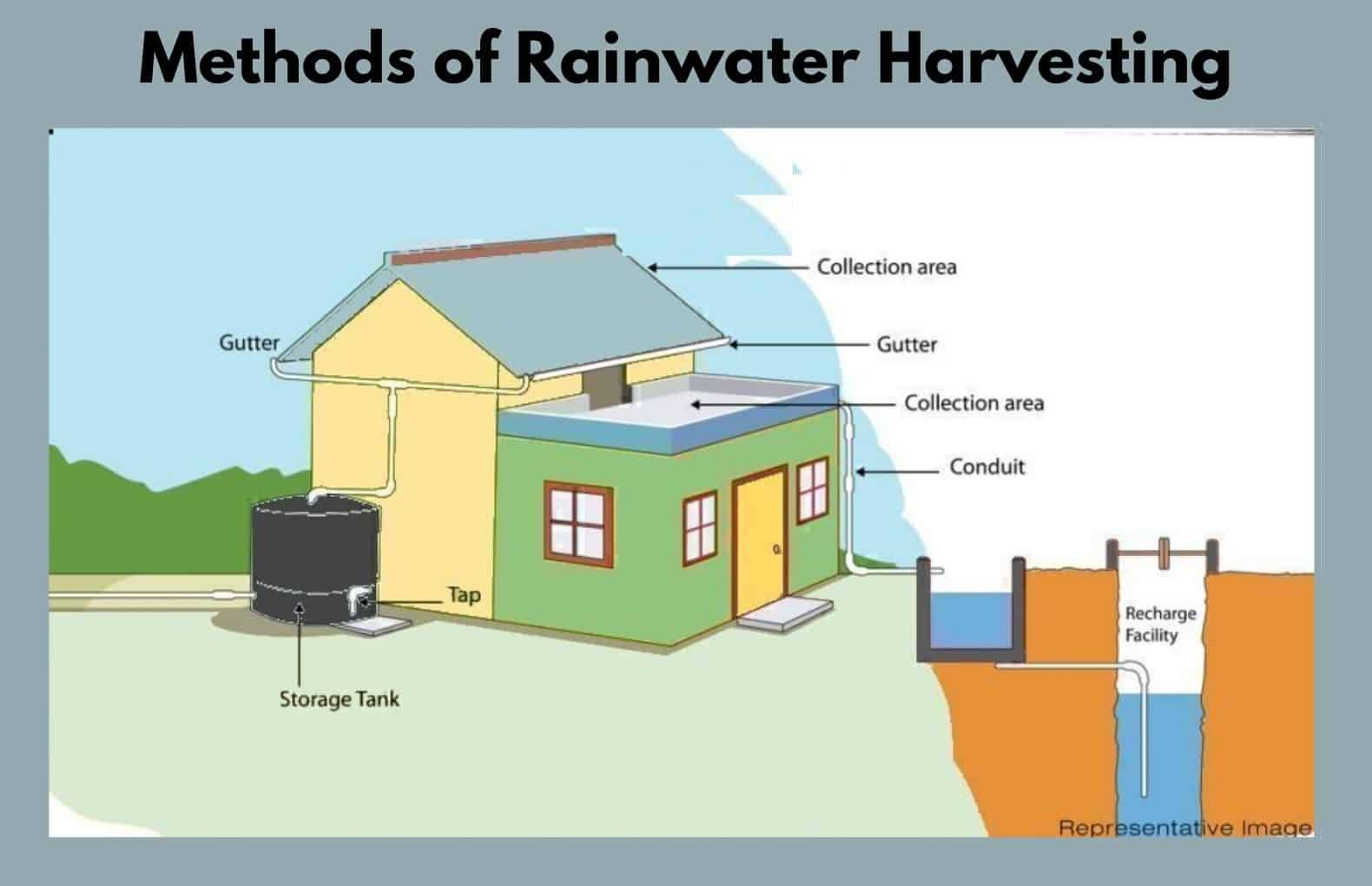Water Cement Ratio | Watercement Ratio | Water Cement Ratio Calculation Example | W/C Ratio Formula4 min read

Post Contents

What Is Water Cement Ratio?

It Is the ratio of the mass of water to the mass of cement added to concrete. The Watercement Ratio directly affects the strength of the concrete mix. The typical w/c ratio for different grades of concrete varies between 0.40 to 0.60.

Importance of Watercement Ratio

In concrete, the most important ingredient that influences that property of concrete is cement and water.

In concrete, the ratio of the weight of water to the weight of cement is called the water to cement ratio (w/c). The cement and water are the two main ingredients responsible for binding everything together.

The water-cement ratio is the ultimate factor for the strength and durability of the concrete when it is cured properly.

For example, if the W/C ratio is 0.40 that’s meant for every 100 lbs of cement used in the concrete, 40 lbs of water is added.

What Happens? If You Add Too Much Water

Adding too much water to concrete can result in shrinkage, cracking, and reduced overall strength. Excessive water makes loose concrete difficult to handle, leads to segregation and bleeding problems in concrete.

What Happens? If You Add Less Water

However, without enough water, it will make concrete to stiff, difficult to fill and compact and it can also result in honeycombing in concrete. it increases the labor effort to move the concrete.

How to calculate the proper amount of water required for concrete?

With the proper water-cement ratio concrete becomes more durability, porosity, and color will be balanced.

Recommended Water cement ratio for concrete,

• For ordinary concrete work (sidewalks and driveways): 0.6 to 0.7
• If required higher quality concrete is desired: 0.4

The practical best water-cement ratio is from about 0.40 to over 0.60.

• A ratio of 0.3 is made concrete too stiff (unless superplasticizers are used).
• A ratio of 0.8 makes concrete wet and fairly weak concrete.

Normal compressive strengths when concrete is properly cured are:

• 0.4 w/c ratio –> 5600 psi
• 0.8 w/c ratio –> 2000 psi.

Water-Cement Ratio Table

The following table indicate the water cement ratio for different grades of concrete as per exposure condition,

Water Cement Ratio Formula

The formula of water cement ratio calculation is water/cement. In simple words, water quantity is divided by cement quantity and result of calculation gives the w/c ratio.

It can be also calculated from Water Cement Ratio IS 10262 (2009) Code Chart

Water Cement Ratio Calculation

For example, if the water-cement ratio is 0.50 for concrete and cement is added is about 50 kg (weight of 1 bag of cement)

Water required for concrete will be,

Water/ cement = 0.50

Water / 50 kg = 0.50

Water = 0.50 x 50

Water = 25 liters = 6.60 gallon

Similarly for W/C = 0.40

Water = 0.40 x 50

Water = 20 liters = 5.28 gallon

As you see that there is water reduced as we decrease the water-cement ratio. It is a fact that water reduced in concrete increases its strength. But there is some limit to the water-cement ratio. The minimum water-cement ratio is 0.30 to 0.35, beyond this concrete becomes too stiff and impractical to handle.

FAQs: Water Cement Ratio

Water Cement Ratio

It Is the ratio of the mass of water to the mass of cement added to concrete. The water cement ratio directly affects the strength of the concrete mix. The typical w/c ratio for different grades of concrete varies between 0.40 to 0.60.

How to Calculate Water Cement Ratio

For example, if the w/c ratio is 0.50 for concrete and cement is added is about 50 kg (weight of 1 bag of cement)
Water required for concrete will be,
Water/ cement = 0.50
Water = 0.50 x 50
Water = 25 liters = 6.60 gallon

Cement Water Ratio

Water Cement Ration is the ratio of the mass of water to the mass of cement added to concrete. The w/c ratio directly affects the strength of the concrete mix. The typical water cement ratio for different grades of concrete varies between 0.40 to 0.60.

You May Also Like:

Popular Post on Website10 Different Types of Ladders and Their Uses | Best Types of Ladders | Material Used For Ladders17 Types Of Contractors | What Is Contractor | 17 Different Types of Contractors | List of Contractors13 Types of Door Hinges | Types of Hinges Used For Door | Different Types Of Door Hinges | What Is A Butt Hinge40 Types of Bridges | Classification of Bridges | 40 Different Types of Bridges Used In The WorldPlumbing Trap | 14 Plumbing Trap Types | Types of Traps In Plumbing | Gully Trap | Types of Drain Traps | Different Types of Traps25 Types of Doors for Your Perfect Home | Type of Door | Type of Doors | Different Types of Doors | Door TypesWhat Is Landscaping | Types of Landscaping Works | Landscaping Meaning | Essential Features of Landscaping WorksWhat Is A Manhole | Purpose of Manhole | Types of Manholes | Manhole Construction DetailsWhat Is Calacatta Quartz | Calacatta Quartz Countertop | Types of Calacatta Quartz | Colours of Calacatta Quartz | Calacatta Quartz Price | Calacatta Quartz Price Per Square Feet﻿
x

Related Posts

What Is Plastering | Plastering Calculation | How to Calculate the Cement Sand Quantity for Plastering | Plastering Ratio
What Is Plastering? Plastering is a method of applying a thin coat or covering of cement mortar on the surface of the brick masonry wall or c...
Setting Time of Cement | Initial and Final Setting Time of Cement Test | Initial Setting Time of Cement | Final Setting Time of Cement
What Is Setting Time of Cement? Initial setting time gives an idea about how fast cement can start losing its plasticity and the final settin...
Foundation Materials List | House Foundation Materials | Materials Used In Foundation Construction
There are different types of Building Foundation Materials used to the foundation of the house. House foundation materials have prime importance...